Gamedev Framework (gf)  0.6.0 A C++11 framework for 2D games
gf::Ease Class Reference

Predefined easing functions. More...

#include <gf/Easings.h>

## Public Member Functions

Ease ()=delete
Deleted constructor. More...

## Static Public Member Functions

static float linear (float t)
Linear easing. More...

static float smooth (float t)
Smooth easing. More...

static float smoother (float t)
Smoother easing. More...

Ease-In flavor of quad easing. More...

Ease-Out flavor of quad easing. More...

Ease-In-Out flavor of quad easing. More...

Ease-Out-In flavor of quad easing. More...

static float cubicIn (float t)
Ease-In flavor of cubic easing. More...

static float cubicOut (float t)
Ease-Out flavor of cubic easing. More...

static float cubicInOut (float t)
Ease-In-Out flavor of cubic easing. More...

static float cubicOutIn (float t)
Ease-Out-In flavor of cubic easing. More...

static float quartIn (float t)
Ease-In flavor of quart easing. More...

static float quartOut (float t)
Ease-Out flavor of quart easing. More...

static float quartInOut (float t)
Ease-In-Out flavor of quart easing. More...

static float quartOutIn (float t)
Ease-Out-In flavor of quart easing. More...

static float quintIn (float t)
Ease-In flavor of quint easing. More...

static float quintOut (float t)
Ease-Out flavor of quint easing. More...

static float quintInOut (float t)
Ease-In-Out flavor of quint easing. More...

static float quintOutIn (float t)
Ease-Out-In flavor of quint easing. More...

static float circIn (float t)
Ease-In flavor of circ easing. More...

static float circOut (float t)
Ease-Out flavor of circ easing. More...

static float circInOut (float t)
Ease-In-Out flavor of circ easing. More...

static float circOutIn (float t)
Ease-Out-In flavor of circ easing. More...

static float sineIn (float t)
Ease-In flavor of sine easing. More...

static float sineOut (float t)
Ease-Out flavor of sine easing. More...

static float sineInOut (float t)
Ease-In-Out flavor of sine easing. More...

static float sineOutIn (float t)
Ease-Out-In flavor of sine easing. More...

static float backIn (float t)
Ease-In flavor of back easing. More...

static float backOut (float t)
Ease-Out flavor of back easing. More...

static float backInOut (float t)
Ease-In-Out flavor of back easing. More...

static float backOutIn (float t)
Ease-Out-In flavor of back easing. More...

static float bounceIn (float t)
Ease-In flavor of bounce easing. More...

static float bounceOut (float t)
Ease-Out flavor of bounce easing. More...

static float bounceInOut (float t)
Ease-Out-In flavor of bounce easing. More...

static float bounceOutIn (float t)
Ease-In-Out flavor of bounce easing. More...

static float elasticIn (float t)
Ease-In flavor of elastic easing. More...

static float elasticOut (float t)
Ease-Out flavor of elastic easing. More...

static float elasticInOut (float t)
Ease-In-Out flavor of elastic easing. More...

static float elasticOutIn (float t)
Ease-Out-In flavor of elastic easing. More...

static float expoIn (float t)
Ease-In flavor of expo easing. More...

static float expoOut (float t)
Ease-Out flavor of expo easing. More...

static float expoInOut (float t)
Ease-In-Out flavor of expo easing. More...

static float expoOutIn (float t)
Ease-Out-In flavor of expo easing. More...

## Detailed Description

Predefined easing functions.

This class defines usual easing functions.

# First set of easing functions

The first set of functions are common interpolation functions.

• linear

$f(t) = t$

• smooth

$f(t) = -2 * t^3 + 3 * t^2$

• smoother

$f(t) = 6 * t^5 - 15 * t^4 + 10 * t^3$

Here are the plots of these functions:

Functions Plot
linearsmooth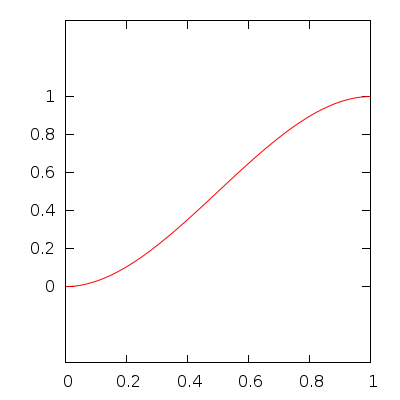smoother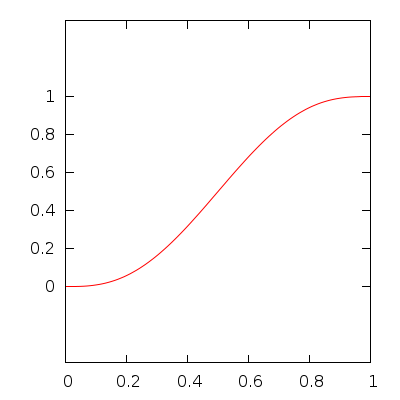# Second set of easing functions

## Definitions

The second set are the easing functions defined by Robert Penner. From a base function $$f(t)$$, four flavors are defined:

• Ease-In: start slow and speed up at the end

$\mathtt{easeIn}(t) = f(t)$

• Ease-Out: start fast and slow down at the end

$\mathtt{easeOut}(t) = 1 - \mathtt{easeIn}(1 - t)$

• Ease-In-Out: start slow, then speed up in the middle and finally slow down at the end

$\mathtt{easeInOut}(t) = \begin{cases} \mathtt{easeIn}(2 \times t) \div 2 & \text{if } t < 0.5 \\ \mathtt{easeOut}(2 \times t + 1) \div 2 + 0.5 & \text{if } t \geq 0.5 \end{cases}$

• Ease-Out-In: start fast, then slow down in the middle and finally speed up at the end

$\mathtt{easeOutIn}(t) = \begin{cases} \mathtt{easeOut}(2 \times t) \div 2 & \text{if } t < 0.5 \\ \mathtt{easeIn}(2 \times t + 1) \div 2 + 0.5 & \text{if } t \geq 0.5 \end{cases}$

## Base functions

Here are the base functions:

$f(t) = t^2$

• cubic

$f(t) = t^3$

• quart

$f(t) = t^4$

• quint

$f(t) = t^5$

• sine

$f(t) = 1 - \cos\left(t \times \frac{\pi}{2}\right)$

• expo

$f(t) = \begin{cases} 0 & \text{if } t = 0 \\ 2^{10 \times (t - 1)} & \text{if } t > 0 \end{cases}$

• circ

$f(t) = 1 - \sqrt{1 - t^2}$

• back

$f(t) = t^2 \times (2.70158 \times t - 1.70158)$

• bounce

$f(t) = \begin{cases} 1 - 7.5625 \times (1 - t)^2 & \text{if } 1 - t < 1 / 2.75 \\ 1 - (7.5625 \times (1 - t - 1.5 / 2.75)^2 + 0.75) & \text{if } 1 - t < 2 / 2.75 \\ 1 - (7.5625 \times (1 - t - 2.25 / 2.75)^2 + 0.9375) & \text{if } 1 - t < 2.5 / 2.75 \\ 1 - (7.5625 \times (1 - t - 2.625 / 2.75)^2 + 0.984375) & \text{otherwise} \end{cases}$

• elastic

$f(t) = -2^{10 \times (t - 1)} \times \sin\left(\left((t - 1) - \frac{0.3}{4}\right) \times \frac{2 \times \pi}{0.3}\right)$

## Plots

Here are the plots for all the defined easing functions:

Function Ease-In Ease-Out Ease-In-Out Ease-Out-In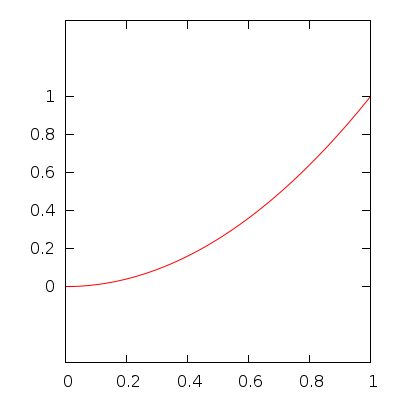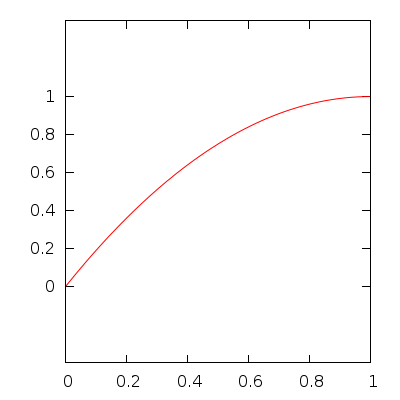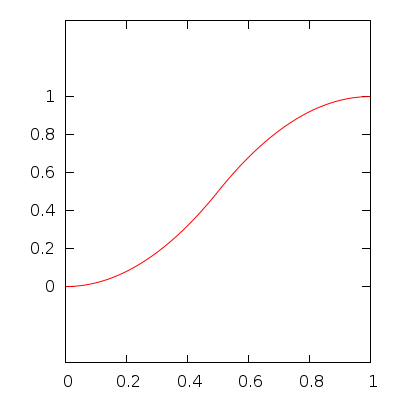cubic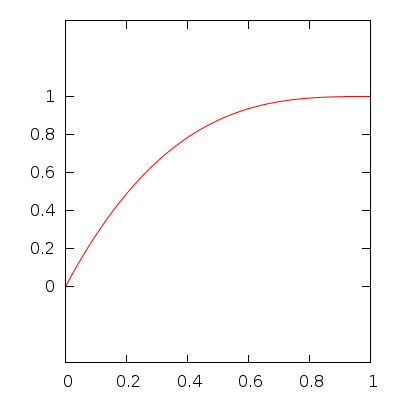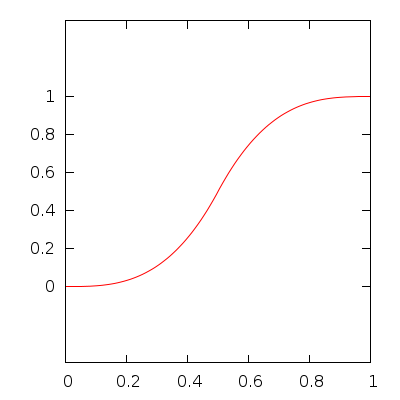quart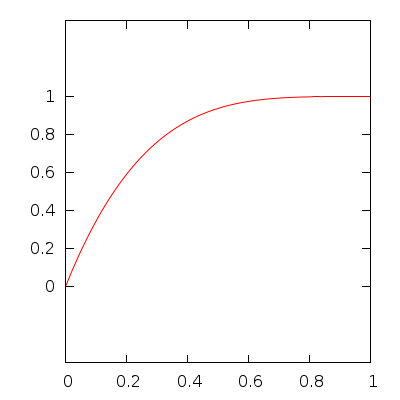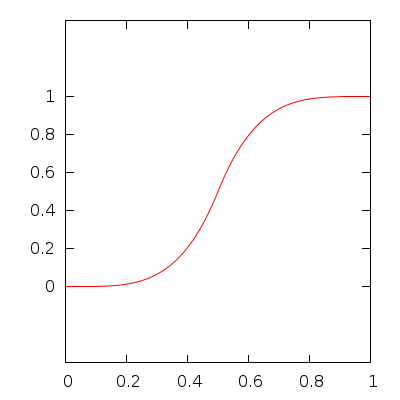quint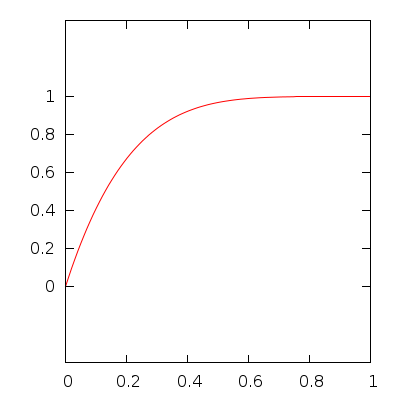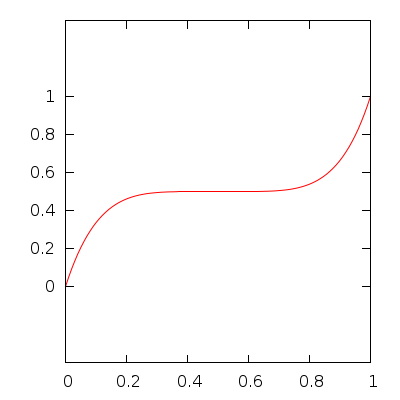sineexpo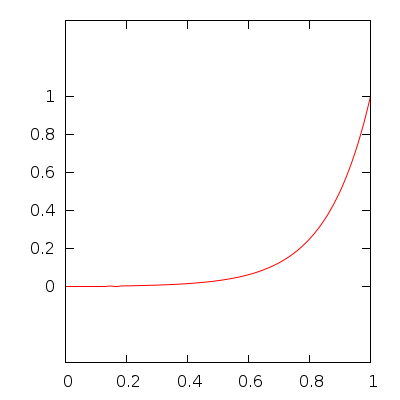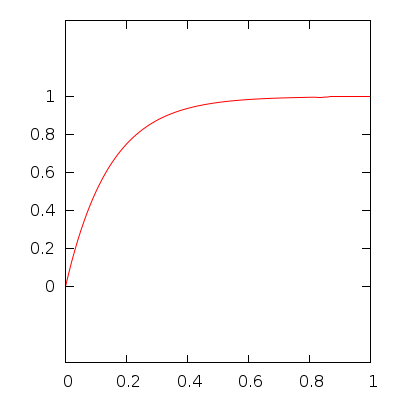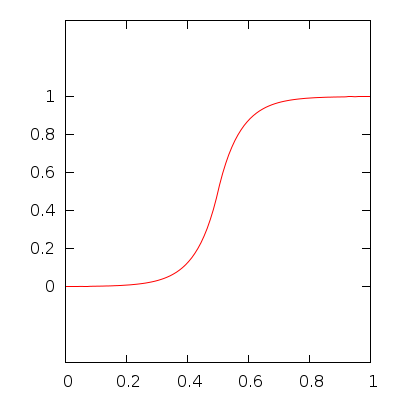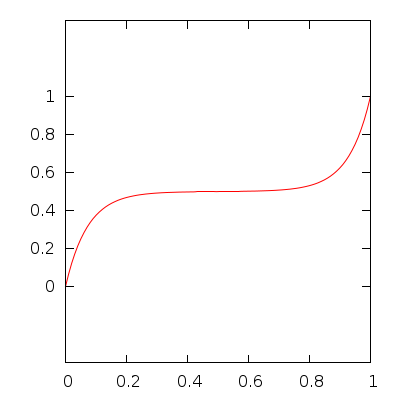circ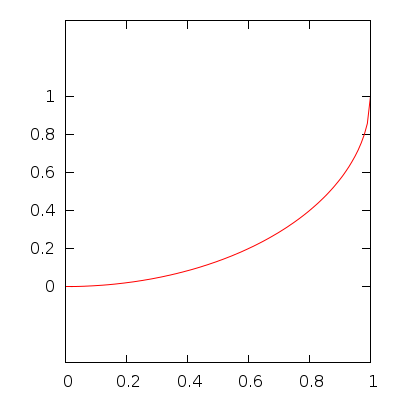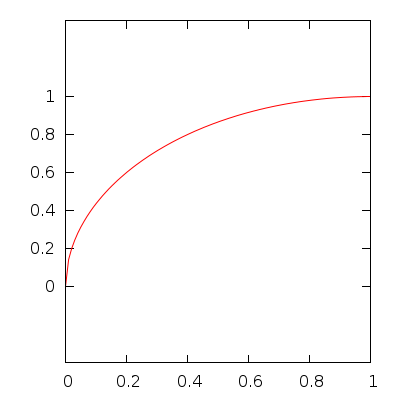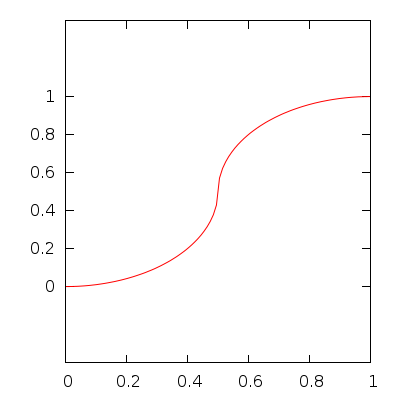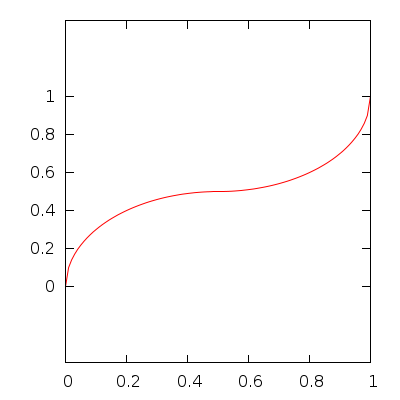back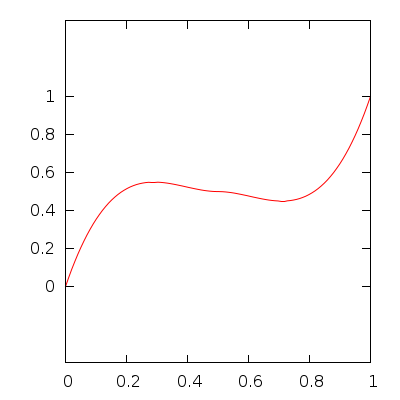bounce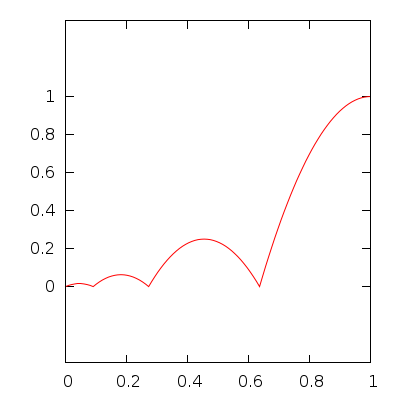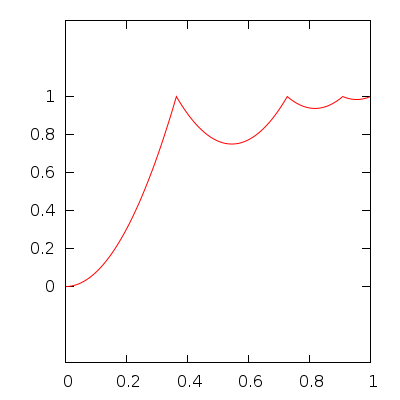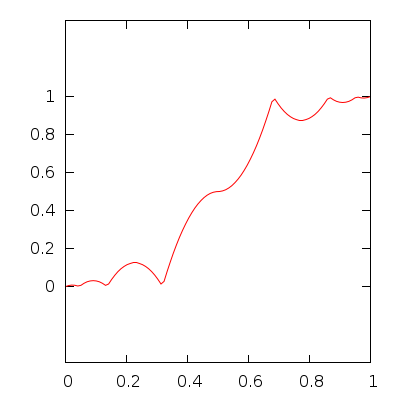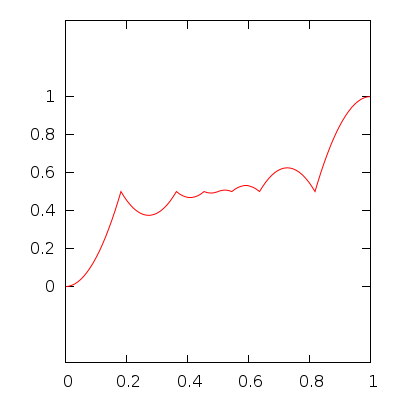elastic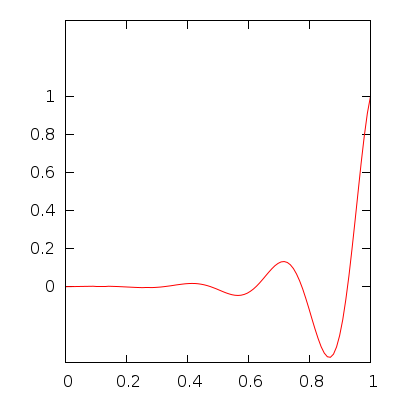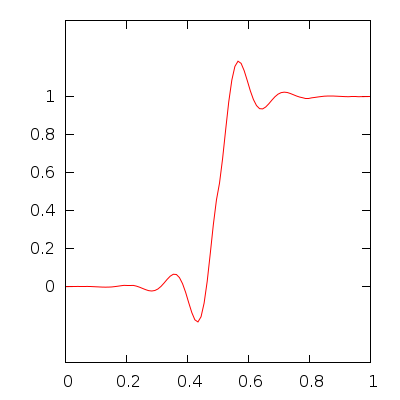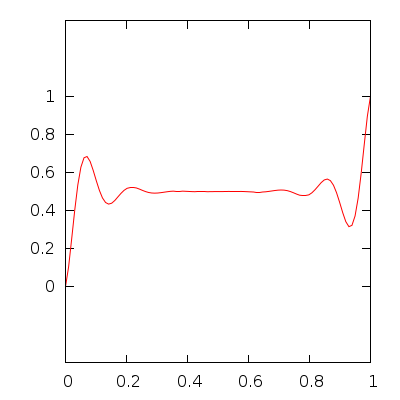gf::Easing
Robert Penner's Easing Functions
Easing Functions Cheat Sheet

## ◆ Ease()

 gf::Ease::Ease ( )
delete

Deleted constructor.

## ◆ backIn()

 static float gf::Ease::backIn ( float t )
static

Ease-In flavor of back easing.

## ◆ backInOut()

 static float gf::Ease::backInOut ( float t )
static

Ease-In-Out flavor of back easing.

## ◆ backOut()

 static float gf::Ease::backOut ( float t )
static

Ease-Out flavor of back easing.

## ◆ backOutIn()

 static float gf::Ease::backOutIn ( float t )
static

Ease-Out-In flavor of back easing.

## ◆ bounceIn()

 static float gf::Ease::bounceIn ( float t )
static

Ease-In flavor of bounce easing.

## ◆ bounceInOut()

 static float gf::Ease::bounceInOut ( float t )
static

Ease-Out-In flavor of bounce easing.

## ◆ bounceOut()

 static float gf::Ease::bounceOut ( float t )
static

Ease-Out flavor of bounce easing.

## ◆ bounceOutIn()

 static float gf::Ease::bounceOutIn ( float t )
static

Ease-In-Out flavor of bounce easing.

## ◆ circIn()

 static float gf::Ease::circIn ( float t )
static

Ease-In flavor of circ easing.

## ◆ circInOut()

 static float gf::Ease::circInOut ( float t )
static

Ease-In-Out flavor of circ easing.

## ◆ circOut()

 static float gf::Ease::circOut ( float t )
static

Ease-Out flavor of circ easing.

## ◆ circOutIn()

 static float gf::Ease::circOutIn ( float t )
static

Ease-Out-In flavor of circ easing.

## ◆ cubicIn()

 static float gf::Ease::cubicIn ( float t )
static

Ease-In flavor of cubic easing.

## ◆ cubicInOut()

 static float gf::Ease::cubicInOut ( float t )
static

Ease-In-Out flavor of cubic easing.

## ◆ cubicOut()

 static float gf::Ease::cubicOut ( float t )
static

Ease-Out flavor of cubic easing.

## ◆ cubicOutIn()

 static float gf::Ease::cubicOutIn ( float t )
static

Ease-Out-In flavor of cubic easing.

## ◆ elasticIn()

 static float gf::Ease::elasticIn ( float t )
static

Ease-In flavor of elastic easing.

## ◆ elasticInOut()

 static float gf::Ease::elasticInOut ( float t )
static

Ease-In-Out flavor of elastic easing.

## ◆ elasticOut()

 static float gf::Ease::elasticOut ( float t )
static

Ease-Out flavor of elastic easing.

## ◆ elasticOutIn()

 static float gf::Ease::elasticOutIn ( float t )
static

Ease-Out-In flavor of elastic easing.

## ◆ expoIn()

 static float gf::Ease::expoIn ( float t )
static

Ease-In flavor of expo easing.

## ◆ expoInOut()

 static float gf::Ease::expoInOut ( float t )
static

Ease-In-Out flavor of expo easing.

## ◆ expoOut()

 static float gf::Ease::expoOut ( float t )
static

Ease-Out flavor of expo easing.

## ◆ expoOutIn()

 static float gf::Ease::expoOutIn ( float t )
static

Ease-Out-In flavor of expo easing.

## ◆ linear()

 static float gf::Ease::linear ( float t )
static

Linear easing.

This is the default easing function

 static float gf::Ease::quadIn ( float t )
static

 static float gf::Ease::quadInOut ( float t )
static

 static float gf::Ease::quadOut ( float t )
static

 static float gf::Ease::quadOutIn ( float t )
static

## ◆ quartIn()

 static float gf::Ease::quartIn ( float t )
static

Ease-In flavor of quart easing.

## ◆ quartInOut()

 static float gf::Ease::quartInOut ( float t )
static

Ease-In-Out flavor of quart easing.

## ◆ quartOut()

 static float gf::Ease::quartOut ( float t )
static

Ease-Out flavor of quart easing.

## ◆ quartOutIn()

 static float gf::Ease::quartOutIn ( float t )
static

Ease-Out-In flavor of quart easing.

## ◆ quintIn()

 static float gf::Ease::quintIn ( float t )
static

Ease-In flavor of quint easing.

## ◆ quintInOut()

 static float gf::Ease::quintInOut ( float t )
static

Ease-In-Out flavor of quint easing.

## ◆ quintOut()

 static float gf::Ease::quintOut ( float t )
static

Ease-Out flavor of quint easing.

## ◆ quintOutIn()

 static float gf::Ease::quintOutIn ( float t )
static

Ease-Out-In flavor of quint easing.

## ◆ sineIn()

 static float gf::Ease::sineIn ( float t )
static

Ease-In flavor of sine easing.

## ◆ sineInOut()

 static float gf::Ease::sineInOut ( float t )
static

Ease-In-Out flavor of sine easing.

## ◆ sineOut()

 static float gf::Ease::sineOut ( float t )
static

Ease-Out flavor of sine easing.

## ◆ sineOutIn()

 static float gf::Ease::sineOutIn ( float t )
static

Ease-Out-In flavor of sine easing.

## ◆ smooth()

 static float gf::Ease::smooth ( float t )
static

Smooth easing.